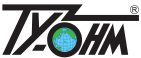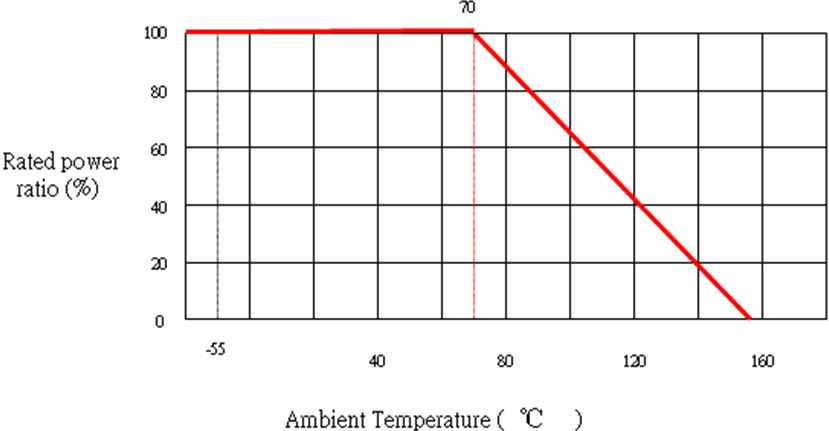Terms and Definitions
1. RATED POWER：

Rated Power is the value of maximum load power specified at rated ambient temperature (usually 70℃).

2. RATED VOLTAGE：

Rated Voltage is the value of maximum DC or AC voltage (rms) specified at rated ambient temperature.  RATED VOLTAGE =3. MAXIMUM WORKING VOLTAGE：

Maximum Working Voltage is the maximum limiting value of above-mentioned Rated Voltage.

Short Time Overload Voltage is the value of allowable overload voltage that can be applied in 5 seconds (Typically 2.5 times of above-mentioned Rated Voltage).

5. DIELECTRIC WITHSTANDING VOLTAGE：

Dielectric Withstanding Voltage is the value of allowable AC voltage (rms) that can be applied between the electrode and the outer coating in 1 minute.

6. DERATING CURVE：

When the ambient temperature surpasses rated ambient temperature, the value of rated  power should be declines as per following DERATING CURVE (usually present as a percentage).7. RESISTANCE TEMPERATURE COEFFICIENT (T.C.R.)：

When the resistors used under different operating ambient temperature, the resistance value will change according to the different operating ambient temperature, usually present in PPM /℃.Hits: 154,179 visits